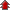# Vector Algebra

• NCERT Chapter### Exercise 1

•  Q1 Represent graphically a displacement of 40 km, 30° east of north. Q2 Classify the following measures as scalars and vectors. (i) 10 kg (ii) 2 metres north-west (iii) 40° (iv) 40 watt (v) 10–19 coulomb (vi) 20 m/s2 Q3 Classify the following as scalar and vector quantities. (i) time period (ii) distance (iii) force (iv) velocity (v) work done Q4 (i) Coinitial (ii) Equal (iii) Collinear but not equal">In Figure, identify the following vectors.(i) Coinitial (ii) Equal (iii) Collinear but not equal Q5 Answer the following as true or false.  \begin{align}(i) \overrightarrow{a}\;  and\; \overrightarrow{-a}\; are\; collinear.\end{align} (ii) Two collinear vectors are always equal in magnitude. (iii) Two vectors having same magnitude are collinear. (iv) Two collinear vectors having the same magnitude are equal.